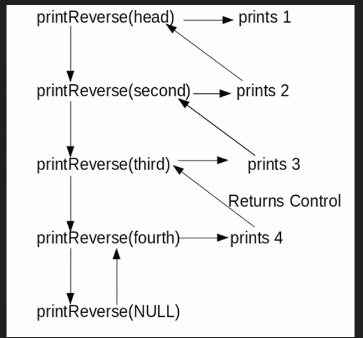Related Articles

# Print reverse of a Linked List without actually reversing

• Difficulty Level : Basic
• Last Updated : 12 May, 2021

Given a linked list, print reverse of it using a recursive function. For example, if the given linked list is 1->2->3->4, then output should be 4->3->2->1.
Note that the question is only about printing the reverse. To reverse the list itself see this
Difficulty Level: RookieAttention reader! Don’t stop learning now. Get hold of all the important DSA concepts with the DSA Self Paced Course at a student-friendly price and become industry ready.  To complete your preparation from learning a language to DS Algo and many more,  please refer Complete Interview Preparation Course.

In case you wish to attend live classes with experts, please refer DSA Live Classes for Working Professionals and Competitive Programming Live for Students.

Algorithm

```printReverse(head)
1. call print reverse for head->next

Implementation:

## C++

 `// C++ program to print reverse of a linked list``#include ``using` `namespace` `std;` `/* Link list node */``class` `Node``{``    ``public``:``    ``int` `data;``    ``Node* next;``};` `/* Function to reverse the linked list */``void` `printReverse(Node* head)``{``    ``// Base case``    ``if` `(head == NULL)``    ``return``;` `    ``// print the list after head node``    ``printReverse(head->next);` `    ``// After everything else is printed, print head``    ``cout << head->data << ``" "``;``}` `/*UTILITY FUNCTIONS*/``/* Push a node to linked list. Note that this function``changes the head */``void` `push(Node** head_ref, ``char` `new_data)``{``    ``/* allocate node */``    ``Node* new_node = ``new` `Node();` `    ``/* put in the data */``    ``new_node->data = new_data;` `    ``/* link the old list off the new node */``    ``new_node->next = (*head_ref);` `    ``/* move the head to pochar to the new node */``    ``(*head_ref) = new_node;``}` `/* Driver code*/``int` `main()``{``    ``// Let us create linked list 1->2->3->4``    ``Node* head = NULL;``    ``push(&head, 4);``    ``push(&head, 3);``    ``push(&head, 2);``    ``push(&head, 1);``    ` `    ``printReverse(head);``    ``return` `0;``}` `// This code is contributed by rathbhupendra`

## C

 `// C program to print reverse of a linked list``#include``#include`` ` `/* Link list node */``struct` `Node``{``    ``int` `data;``    ``struct` `Node* next;``};`` ` `/* Function to reverse the linked list */``void` `printReverse(``struct` `Node* head)``{``    ``// Base case ``    ``if` `(head == NULL)``       ``return``;` `    ``// print the list after head node``    ``printReverse(head->next);` `    ``// After everything else is printed, print head``    ``printf``(``"%d  "``, head->data);``}`` ` `/*UTILITY FUNCTIONS*/``/* Push a node to linked list. Note that this function``  ``changes the head */``void` `push(``struct` `Node** head_ref, ``char` `new_data)``{``    ``/* allocate node */``    ``struct` `Node* new_node =``            ``(``struct` `Node*) ``malloc``(``sizeof``(``struct` `Node));`` ` `    ``/* put in the data  */``    ``new_node->data  = new_data;`` ` `    ``/* link the old list off the new node */``    ``new_node->next = (*head_ref);  `` ` `    ``/* move the head to pochar to the new node */``    ``(*head_ref)    = new_node;``}`` ` `/* Driver program to test above function*/``int` `main()``{``    ``// Let us create linked list 1->2->3->4``    ``struct` `Node* head = NULL;   ``    ``push(&head, 4);``    ``push(&head, 3);``    ``push(&head, 2);``    ``push(&head, 1);``  ` `    ``printReverse(head);``    ``return` `0;``}`

## Java

 `// Java program to print reverse of a linked list``class` `LinkedList``{``    ``Node head;  ``// head of list` `    ``/* Linked list Node*/``    ``class` `Node``    ``{``        ``int` `data;``        ``Node next;``        ``Node(``int` `d) {data = d; next = ``null``; }``    ``}` `    ``/* Function to print reverse of linked list */``    ``void` `printReverse(Node head)``    ``{``        ``if` `(head == ``null``) ``return``;` `        ``// print list of head node``        ``printReverse(head.next);` `        ``// After everything else is printed``        ``System.out.print(head.data+``" "``);``    ``}` `    ``/* Utility Functions */` `    ``/* Inserts a new Node at front of the list. */``    ``public` `void` `push(``int` `new_data)``    ``{``        ``/* 1 & 2: Allocate the Node &``                  ``Put in the data*/``        ``Node new_node = ``new` `Node(new_data);` `        ``/* 3. Make next of new Node as head */``        ``new_node.next = head;` `        ``/* 4. Move the head to point to new Node */``        ``head = new_node;``    ``}` `    ``/*Driver function to test the above methods*/``    ``public` `static` `void` `main(String args[])``    ``{``        ``// Let us create linked list 1->2->3->4``        ``LinkedList llist = ``new` `LinkedList();``        ``llist.push(``4``);``        ``llist.push(``3``);``        ``llist.push(``2``);``        ``llist.push(``1``);` `        ``llist.printReverse(llist.head);``    ``}``}``/* This code is contributed by Rajat Mishra */`

## Python3

 `# Python3 program to print reverse``# of a linked list` `# Node class``class` `Node:``    ` `    ``# Constructor to initialize``    ``# the node object``    ``def` `__init__(``self``, data):``        ` `        ``self``.data ``=` `data``        ``self``.``next` `=` `None``    ` `class` `LinkedList:``    ` `    ``# Function to initialize head``    ``def` `__init__(``self``):``        ``self``.head ``=` `None``    ` `    ``# Recursive function to print``    ``# linked list in reverse order``    ``def` `printrev(``self``, temp):``        ` `        ``if` `temp:``            ``self``.printrev(temp.``next``)``            ``print``(temp.data, end ``=` `' '``)``        ``else``:``            ``return``        ` `    ``# Function to insert a new node``    ``# at the beginning``    ``def` `push(``self``, new_data):``        ` `        ``new_node ``=` `Node(new_data)``        ``new_node.``next` `=` `self``.head``        ``self``.head ``=` `new_node` `# Driver code``llist ``=` `LinkedList()` `llist.push(``4``)``llist.push(``3``)``llist.push(``2``)``llist.push(``1``)` `llist.printrev(llist.head)``    ` `# This code is contributed by Vinay Kumar(vinaykumar71)`

## C#

 `// C# program to print reverse of a linked list``using` `System;` `public` `class` `LinkedList``{``    ``Node head; ``// head of list` `    ``/* Linked list Node*/``    ``class` `Node``    ``{``        ``public` `int` `data;``        ``public` `Node next;``        ``public` `Node(``int` `d)``        ``{``            ``data = d; next = ``null``;``        ``}``    ``}` `    ``/* Function to print reverse of linked list */``    ``void` `printReverse(Node head)``    ``{``        ``if` `(head == ``null``) ``return``;` `        ``// print list of head node``        ``printReverse(head.next);` `        ``// After everything else is printed``        ``Console.Write(head.data + ``" "``);``    ``}` `    ``/* Utility Functions */` `    ``/* Inserts a new Node at front of the list. */``    ``public` `void` `push(``int` `new_data)``    ``{``        ``/* 1 & 2: Allocate the Node &``                ``Put in the data*/``        ``Node new_node = ``new` `Node(new_data);` `        ``/* 3. Make next of new Node as head */``        ``new_node.next = head;` `        ``/* 4. Move the head to point to new Node */``        ``head = new_node;``    ``}` `    ``/*Driver function to test the above methods*/``    ``public` `static` `void` `Main(String []args)``    ``{``        ``// Let us create linked list 1->2->3->4``        ``LinkedList llist = ``new` `LinkedList();``        ``llist.push(4);``        ``llist.push(3);``        ``llist.push(2);``        ``llist.push(1);` `        ``llist.printReverse(llist.head);``    ``}``}` `// This code has been contributed by Rajput-Ji`

## Javascript

 ``

Output:

`4 3 2 1`

Time Complexity: O(n)